# ggExtra - Add marginal histograms to ggplot2, and more ggplot2 enhancements

`ggExtra` is a collection of functions and layers to enhance ggplot2. The flagship function is `ggMarginal`, which can be used to add marginal histograms/boxplots/density plots to ggplot2 scatterplots. You can view a live interactive demo to test it out!

Most other functions/layers are quite simple but are useful because they are fairly common ggplot2 operations that are a bit verbose.

This is an instructional document, but I also wrote a blog post about the reasoning behind and development of this package.

Note: it was brought to my attention that several years ago there was a different package called `ggExtra`, by Baptiste (the author of `gridExtra`). That old `ggExtra` package was deleted in 2011 (two years before I even knew what R is!), and this package has nothing to do with the old one.

## Installation

`ggExtra` is available through both CRAN and GitHub.

To install the CRAN version:

``install.packages("ggExtra")``

To install the latest development version from GitHub:

``````install.packages("devtools")
devtools::install_github("daattali/ggExtra")``````

`ggExtra` comes with an addin for `ggMarginal()`, which lets you interactively add marginal plots to a scatter plot. To use it, simply highlight the code for a ggplot2 plot in your script, and select ggplot2 Marginal Plots from the RStudio Addins menu. Alternatively, you can call the addin directly by calling `ggMarginalGadget(plot)` with a ggplot2 plot.

## Usage

We’ll first load the package and ggplot2, and then see how all the functions work.

``````library("ggExtra")
library("ggplot2")``````

## `ggMarginal` - Add marginal histograms/boxplots/density plots to ggplot2 scatterplots

`ggMarginal()` is an easy drop-in solution for adding marginal density plots/histograms/boxplots to a ggplot2 scatterplot. The easiest way to use it is by simply passing it a ggplot2 scatter plot, and `ggMarginal()` will add the marginal plots.

As a simple first example, let’s create a dataset with 500 points where the x values are normally distributed and the y values are uniformly distributed, and plot a simple ggplot2 scatterplot.

``````set.seed(30)
df1 <- data.frame(x = rnorm(500, 50, 10), y = runif(500, 0, 50))
p1 <- ggplot(df1, aes(x, y)) + geom_point() + theme_bw()
p1``````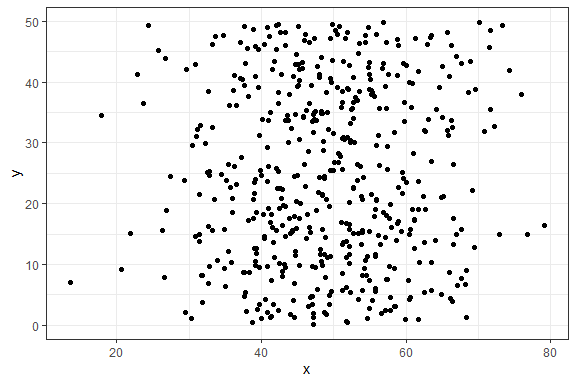And now to add marginal density plots:

``ggMarginal(p1)``That was easy. Notice how the syntax does not follow the standard ggplot2 syntax - you don’t “add” a ggMarginal layer with `p1 + ggMarginal()`, but rather ggMarginal takes the object as an argument and returns a different object. This means that you can use magrittr pipes, for example `p1 %>% ggMarginal()`.

Let’s make the text a bit larger to make it easier to see.

``ggMarginal(p1 + theme_bw(30) + ylab("Two\nlines"))``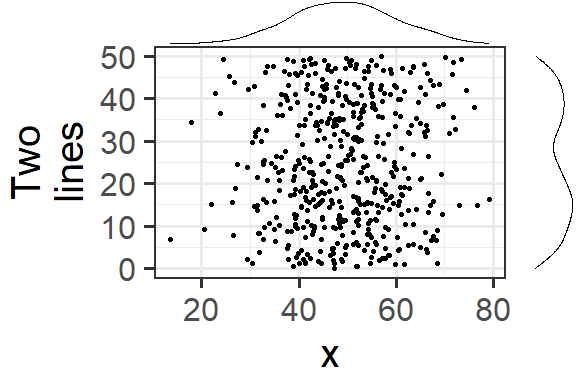Notice how the marginal plots occupy the correct space; even when the main plot’s points are pushed to the right because of larger text or longer axis labels, the marginal plots automatically adjust.

If your scatterplot has a factor variable mapping to a colour (ie. points in the scatterplot are colour-coded according to a variable in the data, by using `aes(colour = ...)`), then you can use `groupColour = TRUE` and/or `groupFill = TRUE` to reflect these groupings in the marginal plots. The result is multiple marginal plots, one for each colour group of points. Here’s an example using the iris dataset.

``````piris <- ggplot(iris, aes(Sepal.Length, Sepal.Width, colour = Species)) +
geom_point()
ggMarginal(piris, groupColour = TRUE, groupFill = TRUE)``````You can also show histograms instead.

``ggMarginal(p1, type = "histogram")``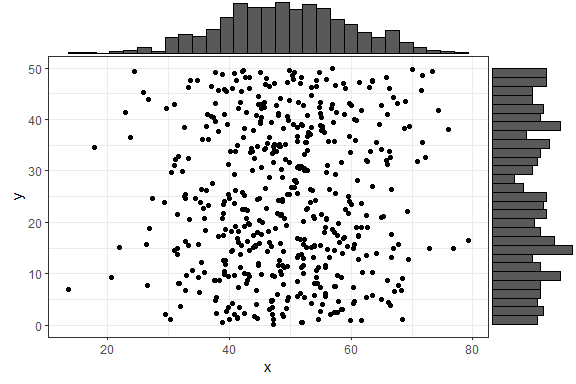There are several more parameters, here is an example with a few more being used. Note that you can use any parameters that the `geom_XXX()` layers accept, such as `col` and `fill`, and they will be passed to these layers.

``````ggMarginal(p1, margins = "x", size = 2, type = "histogram",
col = "blue", fill = "orange")``````In the above example, `size = 2` means that the main scatterplot should occupy twice as much height/width as the margin plots (default is 5). The `col` and `fill` parameters are simply passed to the ggplot layer for both margin plots.

If you want to specify some parameter for only one of the marginal plots, you can use the `xparams` or `yparams` parameters, like this:

``ggMarginal(p1, type = "histogram", xparams = list(binwidth = 1, fill = "orange"))``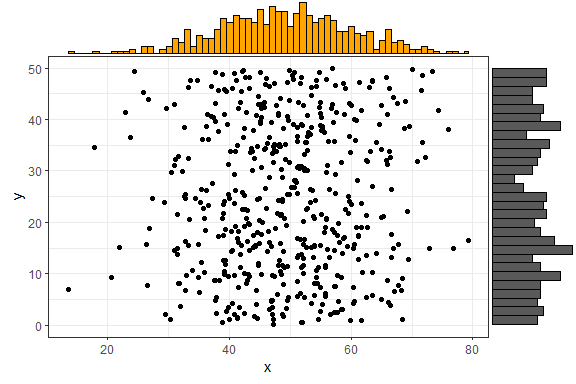Last but not least - you can also save the output from `ggMarginal()` and display it later. (This may sound trivial, but it was not an easy problem to solve - see this discussion).

``````p <- ggMarginal(p1)
p``````You can also create marginal box plots and violin plots. For more information, see `?ggExtra::ggMarginal`.

#### Using `ggMarginal()` in R Notebooks or Rmarkdown

If you try including a `ggMarginal()` plot inside an R Notebook or Rmarkdown code chunk, you’ll notice that the plot doesn’t get output. In order to get a `ggMarginal()` to show up in an these contexts, you need to save the ggMarginal plot as a variable in one code chunk, and explicitly print it using the `grid` package in another chunk, like this:

`````````{r}
library(ggplot2)
library(ggExtra)
p <- ggplot(mtcars, aes(wt, mpg)) + geom_point()
p <- ggMarginal(p)
```
```{r}
grid::grid.newpage()
grid::grid.draw(p)
`````````

## `removeGrid` - Remove grid lines from ggplot2

This is just a convenience function to save a bit of typing and memorization. Minor grid lines are always removed, and the major x or y grid lines can be removed as well (default is to remove both).

`removeGridX` is a shortcut for `removeGrid(x = TRUE, y = FALSE)`, and `removeGridY` is similarly a shortcut for… .

``````df2 <- data.frame(x = 1:50, y = 1:50)
p2 <- ggplot2::ggplot(df2, ggplot2::aes(x, y)) + ggplot2::geom_point()
p2 + removeGrid()``````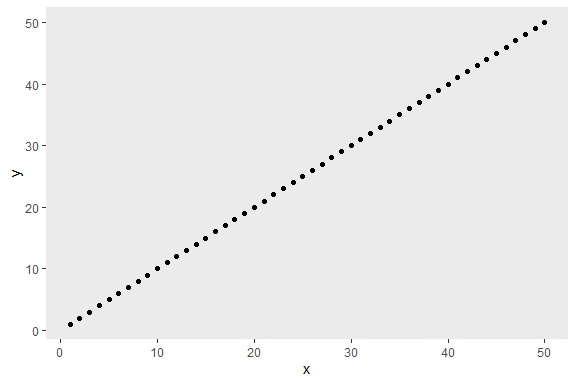For more information, see `?ggExtra::removeGrid`.

## `rotateTextX` - Rotate x axis labels

Often times it is useful to rotate the x axis labels to be vertical if there are too many labels and they overlap. This function accomplishes that and ensures the labels are horizontally centered relative to the tick line.

``````df3 <- data.frame(x = paste("Letter", LETTERS, sep = "_"),
y = seq_along(LETTERS))
p3 <- ggplot2::ggplot(df3, ggplot2::aes(x, y)) + ggplot2::geom_point()
p3 + rotateTextX()``````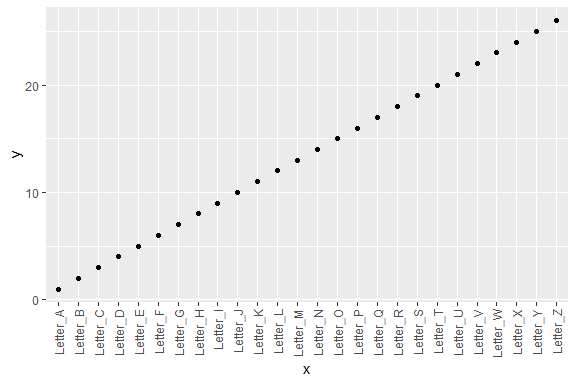For more information, see `?ggExtra::rotateTextX`.

## `plotCount` - Plot count data with ggplot2

This is a convenience function to quickly plot a bar plot of count (frequency) data. The input must be either a frequency table (obtained with `base::table`) or a data.frame with 2 columns where the first column contains the values and the second column contains the counts.

An example using a table:

``plotCount(table(infert\$education))``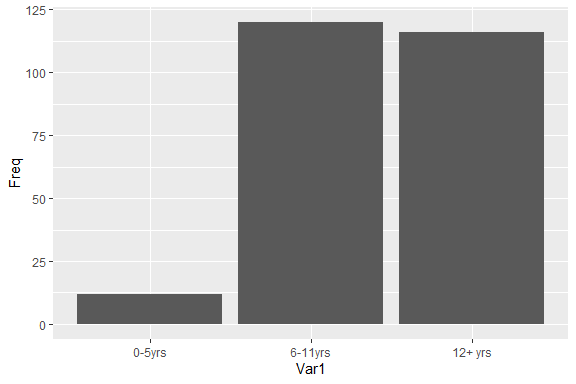An example using a data.frame:

``````df4 <- data.frame("vehicle" = c("bicycle", "car", "unicycle", "Boeing747"),
"NumWheels" = c(2, 4, 1, 16))
plotCount(df4) + removeGridX()``````For more information, see `?ggExtra::plotCount`.